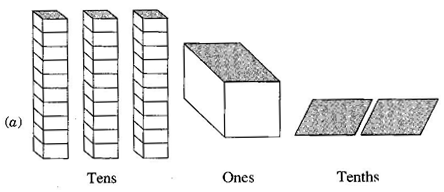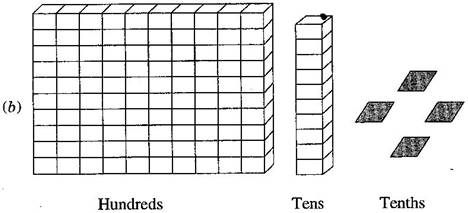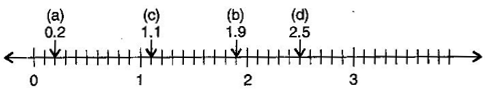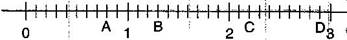### Decimals - Solutions

CBSE Class –VI Mathematics

NCERT Solutions
Chaper 8 Decimals (Ex. 8.1)

Question 1. Write the following as numbers in the given table:Hundreds(100) Tens(10) Ones(1) Tenths(110)$\left(\frac{1}{10}\right)$

 Hundreds(100) Tens(10) Ones(1) Tenths(110)$\left(\frac{1}{10}\right)$ Number 0 3 1 2 31.2 1 1 0 4 110.4

Question 2. Write the following decimals in the place value table:

(a) 19.4

(b) 0.3

(c) 10.6

(d) 205.9

 Hundreds Tens Once Tenths 0 1 9 4

(b)

 Hundreds Tens Once Tenths 0 0 0 3

(c)

 Hundreds Tens Once Tenths 0 1 0 6

(d)

 Hundreds Tens Once Tenths 2 0 5 9

Question 3. Write each of the following as decimals:

(a) seven-tenths

(b) Two tens and nine-tenths

(c) Fourteen point six

(d) One hundred and two-ones

(e) Six hundred point eight

Answer: (a) seven-tenths = 7 tenths = $\frac{7}{10}$ = 0.7

(b) 2 tens and 9-tenths = 2 x 10 + $\frac{9}{10}$ = 20 + 0.9 =20.9

(c) Fourteen point six = 14.6

(d) One hundred and 2-ones = 100 + 2 x 1 = 100 + 2 = 102

(e) Six hundred point eight = 600.8

Question 4. Write each of the following as decimals:

(a) $\frac{5}{10}$

(b) $3+\frac{7}{10}$

(c) $200+60+5+\frac{1}{10}$

(d) $70+\frac{8}{10}$

(e) $\frac{88}{10}$

(f) $4\frac{2}{10}$

(g) $\frac{3}{2}$

(h) $\frac{2}{5}$

(i) $\frac{12}{5}$

(j) $3\frac{3}{5}$

(k) $4\frac{1}{2}$

Answer: (a) $\frac{5}{10}$ = 0.5

(b) $3+\frac{7}{10}$ = 3 + 0.7 = 3.7

(c) $200+60+5+\frac{1}{10}$ = 200 + 60 + 5 + 0.1 = 265.1

(d) $70+\frac{8}{10}$ = 70 + 0.8 = 70.8

(e) $\frac{88}{10}=\frac{80+8}{10}=\frac{80}{10}+\frac{8}{10}=8+\frac{8}{10}$= 8 + 0.8 = 8.8

(f) $4\frac{2}{10}=4+\frac{2}{10}$ = 4 + 0.2 = 4.2

(g) $\frac{3}{2}=\frac{3×5}{2×5}=\frac{15}{10}=\frac{10+5}{10}=\frac{10}{10}+\frac{5}{10}$ = 1 + 0.5 = 1.5

(h) $\frac{2}{5}=\frac{2×2}{5×2}=\frac{4}{10}$ = 0.4

(i) $\frac{12}{5}=\frac{12×2}{5×2}=\frac{24}{10}=\frac{20+4}{10}=\frac{20}{10}+\frac{4}{10}$ = 2 + 0.4 = 2.4

(j) $3\frac{3}{5}=3+\frac{3}{5}=3+\frac{3×2}{5×2}=3+\frac{6}{10}$ = 3 + 0.6 = 3.6

(k) $4\frac{1}{2}=4+\frac{1}{2}=4+\frac{1×5}{2×5}=4+\frac{5}{10}$ = 4 + 0.5 = 4.5

Question 5. Write the following decimals as fraction. Reduce the fractions to lowest terms:

(a) 0.6

(b) 2.5

(c) 1.0

(d) 3.8

(e) 13.7

(f) 21.2

(g) 6.4

Answer: (a) 0.6 = $\frac{6}{10}=\frac{3}{5}$

(b) 2.5 = $\frac{25}{10}=\frac{5}{2}$

(c) 1.0 = $\frac{10}{10}$ = 1

(d) 3.8 = $\frac{38}{10}=\frac{19}{5}$

(e) 13.7 = $\frac{137}{10}$

(f) $21.2=\frac{212}{10}$ = $\frac{106}{5}$

(g) 6.4 = $\frac{64}{10}=\frac{32}{5}$

Question 6. Express the following as cm using decimals:

(a) 2 mm

(b) 30 mm

(c) 116 mm

(d) 4 cm 2 mm

(e) 162 mm

(f) 83 mm

Answer: (a) $\because$ 10 mm = 1 cm

$\therefore$ 1 mm = $\frac{1}{10}$ cm

$\therefore$ 2 mm = $\frac{1}{10}$ x 2 = 0.2 cm

(b) $\because$ 10 mm = 1 cm

$\therefore$ 1 mm = $\frac{1}{10}$ cm

$\therefore$ 30 mm = $\frac{1}{10}$ x 30 = 3.0 cm

(c) $\because$ 10 mm = 1 cm

$\therefore$ 1 mm = $\frac{1}{10}$ cm

$\therefore$ 116 mm = $\frac{1}{10}$ x 116 = 11.6 cm

(d) 4 cm + $\frac{2}{10}$ cm [$\because$ 10 mm = 1 cm]

4 + 0.2 = 4.2 cm

(e) $\because$ 10 mm = 1 cm

$\therefore$ 1 mm = $\frac{1}{10}$ cm

$\therefore$ 162 mm = $\frac{1}{10}$ x 162 = 16.2 cm

(f) $\because$ 10 mm = 1 cm

$\therefore$ 1 mm = $\frac{1}{10}$ cm

$\therefore$ 83 mm = $\frac{1}{10}$ x 83 = 8.3 cm

Question 7. Between which two whole numbers on the number line are the given numbers lie? Which of these whole numbers is nearer  to the given number?

(a) 0.8

(b) 5.1

(c) 2.6

(d) 6.4

(e) 9.1

(f) 4.9

Answer: (a) From 0 to 1, 0.8 is nearest to 1.

(b) From 5 to 6, 5.1 is nearest to 5.

(c) From 2 to 3, 2.6 is nearest to 3.

(d) From 6 to 7, 6.4 is nearest to 6.

(e) From 9 to 10, 9.1 is nearest to 9.

(f) From 4 to 5, 4.9 is nearest to 5.

Question 8. Show the following numbers on the number line:

(a) 0.2

(b) 1.9

(c) 1.1

(d) 2.5Question 9. Write the decimal number represented by the points A, B, C, D:Answer: A = 0 + $\frac{8}{10}$ = 0.8

B = 1 + $\frac{3}{10}$ = 1.3

C = 2 + $\frac{2}{10}$ = 2.2

D = 2 + $\frac{9}{10}$ = 2.9

Question 10. (a) The length of Ramesh’s notebook is 9 cm and 5 mm. What will be its length in cm?

(b) The length of a young gram plant is 65 mm. Express its length in cm.

Answer: (a) 9 cm 5 mm = 9 cm + 5 mm = 9 + $\frac{5}{10}$ = 9.5 cm

(b) 65 mm = $\frac{65}{10}$ cm = 6.5 cm